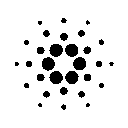Here is the obfuscated code:

``````a=27;b=79;c=C(-2.0,-1.0);d=C(1.0,1.0);e=C(-2.501,-1.003)
newtype C = C (Double,Double) deriving (Show,Eq)
instance Num C where C(x,y)*C(z,t)=C(z*x-y*t,y*z+x*t);C(x,y)+C(z,t)=C(x+z,y+t);abs(C(x,y))=C(sqrt(x*x+y*y),0.0)
r(C(x,y))=x;i(C(x,y))=y
f c z 0=0;f c z n=if(r(abs(z))>2)then n else f c ((z*z)+c) (n-1)
h j k = map (\z->(f (C z) (C(0,0)) 32,(fst z>l - q/2))) [(x,y)|y<-[p,(p+((o-p)/a))..o],x<-[m,(m + q)..l]] where o=i k;p=i j;m=r j;l=r k;q=(l-m)/b
u j k = concat \$ map v \$ h j k where v (i,p)=(" .,`'°\":;-+oO0123456789=!%*§&[email protected]#"!!i):rst p;rst True="\n";rst False=""
main = putStrLn \$ im 0 where cl n (C (x,y))=let cs=(1.1**n-1) in C ((x+cs*(r e))/cs+1,(y+cs*(i e))/cs+1);bl n=cl n c;tr n=cl n d;im n=u (bl n) (tr n)++"\x1b[H\x1b[25A"++im (n+1)``````

To launch it, you’ll need to have haskell installed and to run:

`ghc –make animandel.hs && animandel`

Here is some image after 50 iterations:

```###@@@@@@@\$\$\$\$\$\$\$\$\$\$\$\$\$\$\$\$\$\$\$\$\$\$\$\$\$\$\$\$\$\$\$\$\$\$\$\$\$\$&&&&&WWOOClbUOWW&&\$\$\$\$\$\$\$\$\$\$\$\$\$\$
##@@@@@@\$\$\$\$\$\$\$\$\$\$\$\$\$\$\$\$\$\$\$\$\$\$\$\$\$\$\$\$\$\$\$\$\$\$\$\$\$\$&&&&&WWUCUb; ,jUOWW&&&\$\$\$\$\$\$\$\$\$\$\$\$
#@@@@@\$\$\$\$\$\$\$\$\$\$\$\$\$\$\$\$\$\$\$\$\$\$\$\$\$\$\$\$\$\$\$\$\$\$\$\$\$\$&&&WWWWWUb       ooCWW&&&&&&\$\$\$\$\$\$\$\$
@@@@\$\$\$\$\$\$\$\$\$\$\$\$\$\$\$\$\$\$\$\$\$\$\$\$\$\$\$\$\$\$\$\$\$\$\$\$\$&&WWWWWWWWOU         uUOWWWW&&&&&&\$\$\$\$\$
@@@\$\$\$\$\$\$\$\$\$\$\$\$\$\$\$\$\$\$\$\$\$\$\$\$\$\$\$\$\$\$\$\$\$\$&&&WOUObUOOOUUUCbi      rbCUUUOWWWWWOUW&\$\$\$
@\$\$\$\$\$\$\$\$\$\$\$\$\$\$\$\$\$\$\$\$\$\$\$\$\$\$\$\$\$\$\$&&&&&&WWWUcr,iiCb                o wUUUUUC;OW&\$\$
\$\$\$\$\$\$\$\$\$\$\$\$\$\$\$\$\$\$\$\$\$\$\$\$\$\$&&&&&&&&&&WWWWOUC,                         j    llW&&\$
\$\$\$\$\$\$\$\$\$\$\$\$\$\$\$\$\$\$\$\$\$&&&&&&&&&&&&WWWWWWOCCbi                              bWWW&&
\$\$\$\$\$\$\$\$\$\$\$\$\$\$\$\$\$&&WWWWWWW&&&WWWWWWWWOUo                                 jUOWW&&
\$\$\$\$\$\$\$\$\$\$\$\$\$\$&&&WWOwOOWWWOUUOWWWWWOOUbw                                  j.blW&
\$\$\$\$\$\$\$\$\$\$\$&&&&&WWWObiijbUCl bCiUUUUUCj,                                    bOW&
\$\$\$\$\$\$\$\$\$&&&&&&&WWWOUbw  ;      oobCbl                                     jUWW&
\$\$\$\$\$\$\$&&&&&&&WWWWOcbi             ij                                      jUW&&
\$\$\$\$\$&&WWWWWWWOwUUCbw                                                       WW&&
WWWOWWWWWWWWWUUbo                                                         UWWW&&
:                                                                      wbUOWW&&&
WWWOWWWWWWWWWUUbo                                                         UWWW&&
\$\$\$\$\$&&WWWWWWWOwUUCbw                                                       WW&&
\$\$\$\$\$\$\$&&&&&&&WWWWOcbi             ij                                      jUW&&
\$\$\$\$\$\$\$\$\$&&&&&&&WWWOUbw  ;      oobCbl                                     jUWW&
\$\$\$\$\$\$\$\$\$\$\$&&&&&WWWObiijbUCl bCiUUUUUCj,                                    bOW&
\$\$\$\$\$\$\$\$\$\$\$\$\$\$&&&WWOwOOWWWOUUOWWWWWOOUbw                                  j.blW&
\$\$\$\$\$\$\$\$\$\$\$\$\$\$\$\$\$&&WWWWWWW&&&WWWWWWWWOUo                                 jUOWW&&
\$\$\$\$\$\$\$\$\$\$\$\$\$\$\$\$\$\$\$\$\$&&&&&&&&&&&&WWWWWWOCCbi                              bWWW&&
\$\$\$\$\$\$\$\$\$\$\$\$\$\$\$\$\$\$\$\$\$\$\$\$\$\$&&&&&&&&&&WWWWOUC,                         j    llW&&\$
@\$\$\$\$\$\$\$\$\$\$\$\$\$\$\$\$\$\$\$\$\$\$\$\$\$\$\$\$\$\$\$&&&&&&WWWUcr,iiCb                o wUUUUUC;OW&\$\$
```

Here is the more readable version. I believe with this far more readable version, no more explanation is needed.

``````nbvert = 30
nbhor = 79
zoomfactor = 1.01
init_bottom_left = C (-2.0,-2.0)
init_top_right   = C (3.0,2.0)
interrest        = C (-1.713,-0.000)

newtype Complex = C (Float,Float) deriving (Show,Eq)
instance Num Complex where
fromInteger n     = C (fromIntegral n,0.0)
C (x,y) * C (z,t) = C (z*x - y*t, y*z + x*t)
C (x,y) + C (z,t) = C (x+z, y+t)
abs (C (x,y))     = C (sqrt (x*x + y*y),0.0)
signum (C (x,y))  = C (signum x , 0.0)

real :: Complex -> Float
real (C (x,y))    = x
im :: Complex -> Float
im   (C (x,y))    = y

cabs :: Complex -> Float
cabs = real.abs

f :: Complex -> Complex -> Int -> Int
f c z 0 = 0
f c z n = if (cabs z > 2) then n else f c ((z*z)+c) (n-1)

bmandel bottomleft topright = map (\z -> (f (C z) (C(0,0)) 32, (fst z > right - hstep/2 ))) [(x,y) | y <- [bottom,(bottom + vstep)..top], x<-[left,(left + hstep)..right]]
where
top = im topright
bottom = im bottomleft
left = real bottomleft
right = real topright
vstep=(top-bottom)/nbvert
hstep=(right-left)/nbhor

mandel :: (Complex,Complex) -> String
mandel (bottomleft,topright) = concat \$ map treat \$ bmandel bottomleft topright
where
treat (i,jump) = " .,:;rcuowijlbCUOW&[email protected]#" !! (div (i*22) 32):rst jump
rst True = "\n"
rst False = ""

cdiv :: Complex -> Float -> Complex
cdiv (C(x,y)) r = C(x/r, y/r)
cmul :: Complex -> Float -> Complex
cmul (C(x,y)) r = C(x*r, y*r)

zoom :: Complex -> Complex -> Complex -> Float -> (Complex,Complex)
zoom bl tr center magn = (f bl, f tr)
where
f point = ((center `cmul` magn) + point ) `cdiv` (magn + 1)

main = do
x <- getContents
putStrLn \$ infinitemandel 0
where
window n = zoom init_bottom_left init_top_right interrest (zoomfactor**n)
infinitemandel n = mandel (window n) ++ "\x1b[H\x1b[25A" ++ infinitemandel (n+1)``````
Published on 2011-07-10
Done with Vim spacemacs & nanoc HakyllADA: ``` DdzFFzCqrhtAvdkmATx5Fm8NPJViDy85ZBw13p4XcNzVzvQg8e3vWLXq23JQWFxPEXK6Kvhaxxe7oJt4VMYHxpA2vtCFiP8fziohN6Yp ```